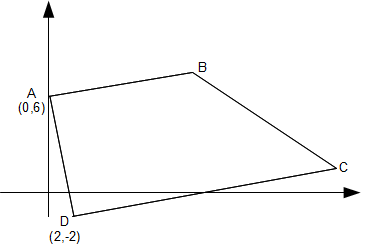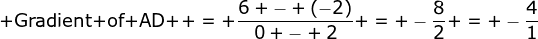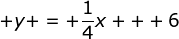### Coordinate Geometry Part I

Technique 1: Finding the gradient of a lineParallel lines
Parallel lines have the same gradient
Perpendicular lines
If a line has a gradient of 1/2 , the gradient of the line perpendicular to it is -2.
-2 is the negative reciprocal of 1/2. In other words, we "flip" the fraction 1/2 to get 2 and attach a negative sign

Technique 2: Finding equation of a straight line
The general format of a straight line is y = mx + c
where m is the gradient and,
c is the y intercept

Example 2
Find the equation of a line that contains the points (1,2) and (3,4).

From the example above we know that gradient of the line is 1.
Hence the equation of the line is: y = 1x + c

To find the y intercept, c, we sub in one of the points
I choose the point (1,2) and sub x =1, y = 2
2 = 1 + c
Hence c = 1

The equation of the line is y = x + 1

Let us combine the techniques we have learnt so far to solve a typical O Level question

Question 1The figure above shows a trapezium where AB is parallel to CD. Both the lines AB and CD are perpendicular to AD. Find the equation of line AB

SolutionSince AB is perpendicular to AD, the gradient of line AB is the negative reciprocal of -4 which is 1/4

Find the y intercept
From the figure we can see that the y intercept, c of the line AB is 6

Find equation of line
Therefore the equation of line AB is